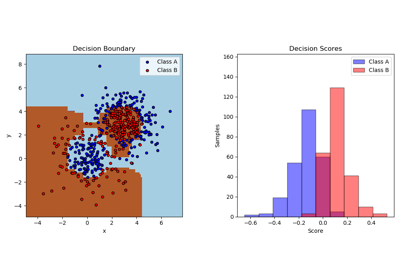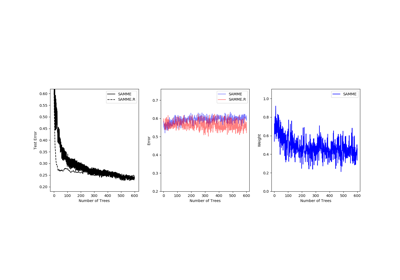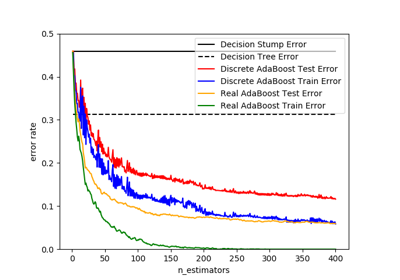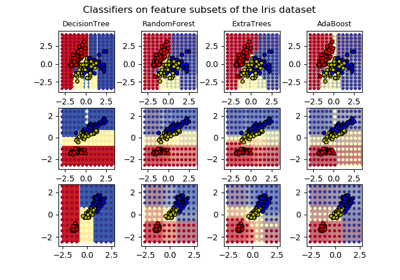# sklearn.ensemble.AdaBoostClassifier¶

class sklearn.ensemble.AdaBoostClassifier(base_estimator=None, n_estimators=50, learning_rate=1.0, algorithm=’SAMME.R’, random_state=None)[source]

An AdaBoost  classifier is a meta-estimator that begins by fitting a classifier on the original dataset and then fits additional copies of the classifier on the same dataset but where the weights of incorrectly classified instances are adjusted such that subsequent classifiers focus more on difficult cases.

This class implements the algorithm known as AdaBoost-SAMME .

Read more in the User Guide.

Parameters: base_estimator : object, optional (default=None) The base estimator from which the boosted ensemble is built. Support for sample weighting is required, as well as proper classes_ and n_classes_ attributes. If None, then the base estimator is DecisionTreeClassifier(max_depth=1) n_estimators : integer, optional (default=50) The maximum number of estimators at which boosting is terminated. In case of perfect fit, the learning procedure is stopped early. learning_rate : float, optional (default=1.) Learning rate shrinks the contribution of each classifier by learning_rate. There is a trade-off between learning_rate and n_estimators. algorithm : {‘SAMME’, ‘SAMME.R’}, optional (default=’SAMME.R’) If ‘SAMME.R’ then use the SAMME.R real boosting algorithm. base_estimator must support calculation of class probabilities. If ‘SAMME’ then use the SAMME discrete boosting algorithm. The SAMME.R algorithm typically converges faster than SAMME, achieving a lower test error with fewer boosting iterations. random_state : int, RandomState instance or None, optional (default=None) If int, random_state is the seed used by the random number generator; If RandomState instance, random_state is the random number generator; If None, the random number generator is the RandomState instance used by np.random. estimators_ : list of classifiers The collection of fitted sub-estimators. classes_ : array of shape = [n_classes] The classes labels. n_classes_ : int The number of classes. estimator_weights_ : array of floats Weights for each estimator in the boosted ensemble. estimator_errors_ : array of floats Classification error for each estimator in the boosted ensemble. feature_importances_ : array of shape = [n_features] Return the feature importances (the higher, the more important the feature).

References

 [R33e4ec8c4ad5-1] Y. Freund, R. Schapire, “A Decision-Theoretic Generalization of on-Line Learning and an Application to Boosting”, 1995.

Examples

>>> from sklearn.ensemble import AdaBoostClassifier
>>> from sklearn.datasets import make_classification
>>> X, y = make_classification(n_samples=1000, n_features=4,
...                            n_informative=2, n_redundant=0,
...                            random_state=0, shuffle=False)
>>> clf.fit(X, y)
learning_rate=1.0, n_estimators=100, random_state=0)
>>> clf.feature_importances_
array([0.28..., 0.42..., 0.14..., 0.16...])
>>> clf.predict([[0, 0, 0, 0]])
array()
>>> clf.score(X, y)
0.983...


Methods

 decision_function(self, X) Compute the decision function of X. fit(self, X, y[, sample_weight]) Build a boosted classifier from the training set (X, y). get_params(self[, deep]) Get parameters for this estimator. predict(self, X) Predict classes for X. predict_log_proba(self, X) Predict class log-probabilities for X. predict_proba(self, X) Predict class probabilities for X. score(self, X, y[, sample_weight]) Returns the mean accuracy on the given test data and labels. set_params(self, \*\*params) Set the parameters of this estimator. staged_decision_function(self, X) Compute decision function of X for each boosting iteration. staged_predict(self, X) Return staged predictions for X. staged_predict_proba(self, X) Predict class probabilities for X. staged_score(self, X, y[, sample_weight]) Return staged scores for X, y.
__init__(self, base_estimator=None, n_estimators=50, learning_rate=1.0, algorithm=’SAMME.R’, random_state=None)[source]
decision_function(self, X)[source]

Compute the decision function of X.

Parameters: X : {array-like, sparse matrix} of shape = [n_samples, n_features] The training input samples. Sparse matrix can be CSC, CSR, COO, DOK, or LIL. COO, DOK, and LIL are converted to CSR. score : array, shape = [n_samples, k] The decision function of the input samples. The order of outputs is the same of that of the classes_ attribute. Binary classification is a special cases with k == 1, otherwise k==n_classes. For binary classification, values closer to -1 or 1 mean more like the first or second class in classes_, respectively.
feature_importances_
Return the feature importances (the higher, the more important the
feature).
Returns: feature_importances_ : array, shape = [n_features]
fit(self, X, y, sample_weight=None)[source]

Build a boosted classifier from the training set (X, y).

Parameters: X : {array-like, sparse matrix} of shape = [n_samples, n_features] The training input samples. Sparse matrix can be CSC, CSR, COO, DOK, or LIL. COO, DOK, and LIL are converted to CSR. y : array-like of shape = [n_samples] The target values (class labels). sample_weight : array-like of shape = [n_samples], optional Sample weights. If None, the sample weights are initialized to 1 / n_samples. self : object
get_params(self, deep=True)[source]

Get parameters for this estimator.

Parameters: deep : boolean, optional If True, will return the parameters for this estimator and contained subobjects that are estimators. params : mapping of string to any Parameter names mapped to their values.
predict(self, X)[source]

Predict classes for X.

The predicted class of an input sample is computed as the weighted mean prediction of the classifiers in the ensemble.

Parameters: X : {array-like, sparse matrix} of shape = [n_samples, n_features] The training input samples. Sparse matrix can be CSC, CSR, COO, DOK, or LIL. COO, DOK, and LIL are converted to CSR. y : array of shape = [n_samples] The predicted classes.
predict_log_proba(self, X)[source]

Predict class log-probabilities for X.

The predicted class log-probabilities of an input sample is computed as the weighted mean predicted class log-probabilities of the classifiers in the ensemble.

Parameters: X : {array-like, sparse matrix} of shape = [n_samples, n_features] The training input samples. Sparse matrix can be CSC, CSR, COO, DOK, or LIL. COO, DOK, and LIL are converted to CSR. p : array of shape = [n_samples, n_classes] The class probabilities of the input samples. The order of outputs is the same of that of the classes_ attribute.
predict_proba(self, X)[source]

Predict class probabilities for X.

The predicted class probabilities of an input sample is computed as the weighted mean predicted class probabilities of the classifiers in the ensemble.

Parameters: X : {array-like, sparse matrix} of shape = [n_samples, n_features] The training input samples. Sparse matrix can be CSC, CSR, COO, DOK, or LIL. COO, DOK, and LIL are converted to CSR. p : array of shape = [n_samples, n_classes] The class probabilities of the input samples. The order of outputs is the same of that of the classes_ attribute.
score(self, X, y, sample_weight=None)[source]

Returns the mean accuracy on the given test data and labels.

In multi-label classification, this is the subset accuracy which is a harsh metric since you require for each sample that each label set be correctly predicted.

Parameters: X : array-like, shape = (n_samples, n_features) Test samples. y : array-like, shape = (n_samples) or (n_samples, n_outputs) True labels for X. sample_weight : array-like, shape = [n_samples], optional Sample weights. score : float Mean accuracy of self.predict(X) wrt. y.
set_params(self, **params)[source]

Set the parameters of this estimator.

The method works on simple estimators as well as on nested objects (such as pipelines). The latter have parameters of the form <component>__<parameter> so that it’s possible to update each component of a nested object.

Returns: self
staged_decision_function(self, X)[source]

Compute decision function of X for each boosting iteration.

This method allows monitoring (i.e. determine error on testing set) after each boosting iteration.

Parameters: X : {array-like, sparse matrix} of shape = [n_samples, n_features] The training input samples. Sparse matrix can be CSC, CSR, COO, DOK, or LIL. COO, DOK, and LIL are converted to CSR. score : generator of array, shape = [n_samples, k] The decision function of the input samples. The order of outputs is the same of that of the classes_ attribute. Binary classification is a special cases with k == 1, otherwise k==n_classes. For binary classification, values closer to -1 or 1 mean more like the first or second class in classes_, respectively.
staged_predict(self, X)[source]

Return staged predictions for X.

The predicted class of an input sample is computed as the weighted mean prediction of the classifiers in the ensemble.

This generator method yields the ensemble prediction after each iteration of boosting and therefore allows monitoring, such as to determine the prediction on a test set after each boost.

Parameters: X : array-like of shape = [n_samples, n_features] The input samples. Sparse matrix can be CSC, CSR, COO, DOK, or LIL. COO, DOK, and LIL are converted to CSR. y : generator of array, shape = [n_samples] The predicted classes.
staged_predict_proba(self, X)[source]

Predict class probabilities for X.

The predicted class probabilities of an input sample is computed as the weighted mean predicted class probabilities of the classifiers in the ensemble.

This generator method yields the ensemble predicted class probabilities after each iteration of boosting and therefore allows monitoring, such as to determine the predicted class probabilities on a test set after each boost.

Parameters: X : {array-like, sparse matrix} of shape = [n_samples, n_features] The training input samples. Sparse matrix can be CSC, CSR, COO, DOK, or LIL. COO, DOK, and LIL are converted to CSR. p : generator of array, shape = [n_samples] The class probabilities of the input samples. The order of outputs is the same of that of the classes_ attribute.
staged_score(self, X, y, sample_weight=None)[source]

Return staged scores for X, y.

This generator method yields the ensemble score after each iteration of boosting and therefore allows monitoring, such as to determine the score on a test set after each boost.

Parameters: X : {array-like, sparse matrix} of shape = [n_samples, n_features] The training input samples. Sparse matrix can be CSC, CSR, COO, DOK, or LIL. COO, DOK, and LIL are converted to CSR. y : array-like, shape = [n_samples] Labels for X. sample_weight : array-like, shape = [n_samples], optional Sample weights. z : float

## Examples using sklearn.ensemble.AdaBoostClassifier¶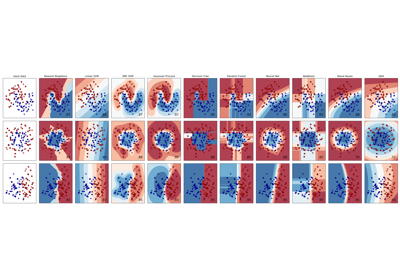Classifier comparison